Working with Sparse Matrices in R

base R has the Matrix package which provides various classes of sparse (and non-sparse) matrix formats and routines for fast matrix math with these matrices. This is handy however a few common tasks are incredibly slow with matrices in Matrix class due to overhead with all the checking and class changing after manipulations.

For example, adding a matrix + a Matrix or Matrix + Matrix is slow.

library(microbenchmark)
## Warning: package 'microbenchmark' was built under R version 3.4.4
library(Matrix)
library(ggplot2)
n=100
A=Matrix(0, n, n) #dsCMatrix, sparse
B=as.matrix(A)
C=Matrix(1:n, n, n) #dgeMatrix, dense
res=microbenchmark(B+B,A+A,A+B,A+C,B+C)
autoplot(res)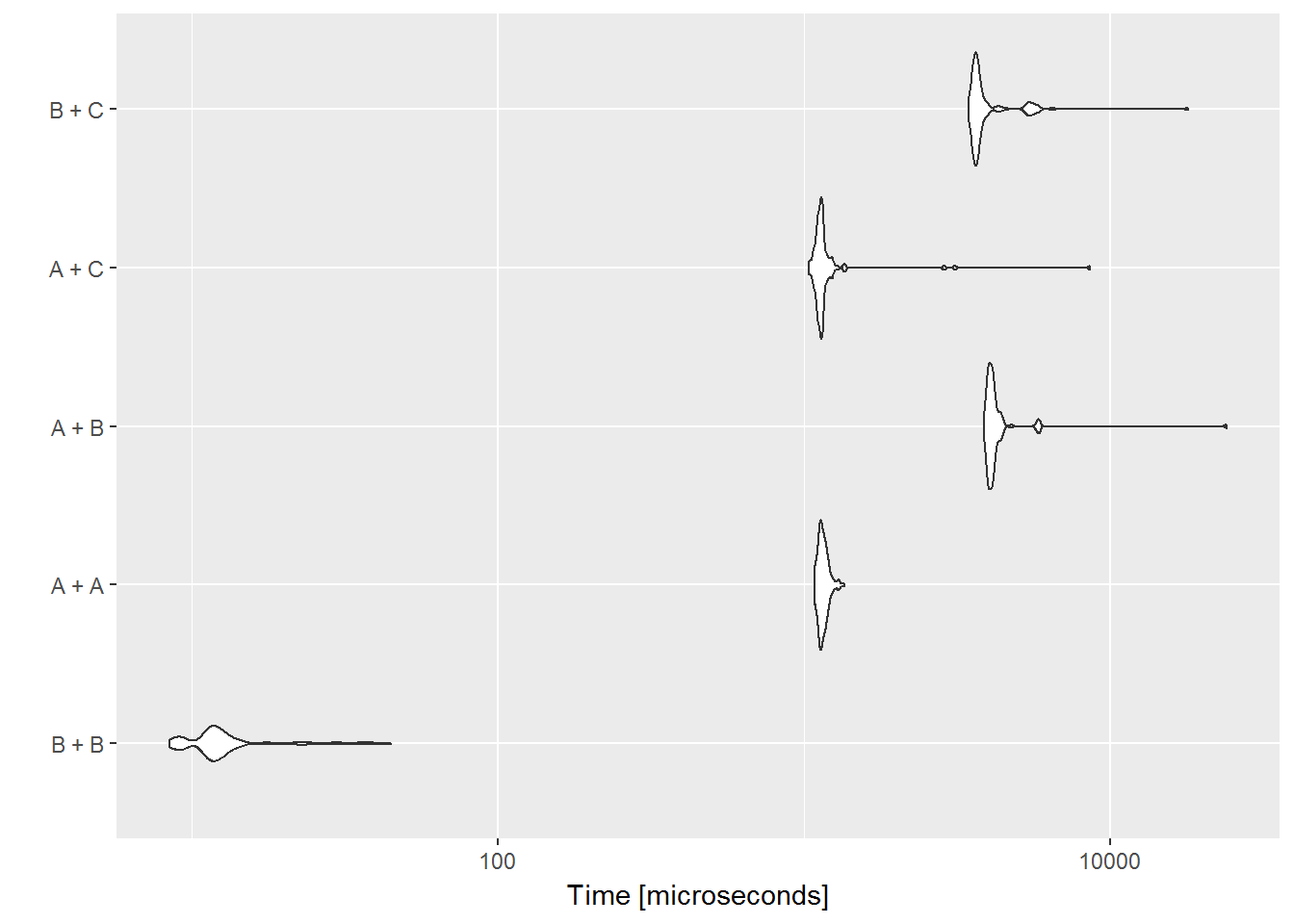As n gets large, A+A will be fine but A+B will remain slow. Obviously as n goes up, the size of A is much smaller than B if A is sparse.

Multiplication is fine.

res=microbenchmark(B%*%B,A%*%B,A%*%A)
autoplot(res)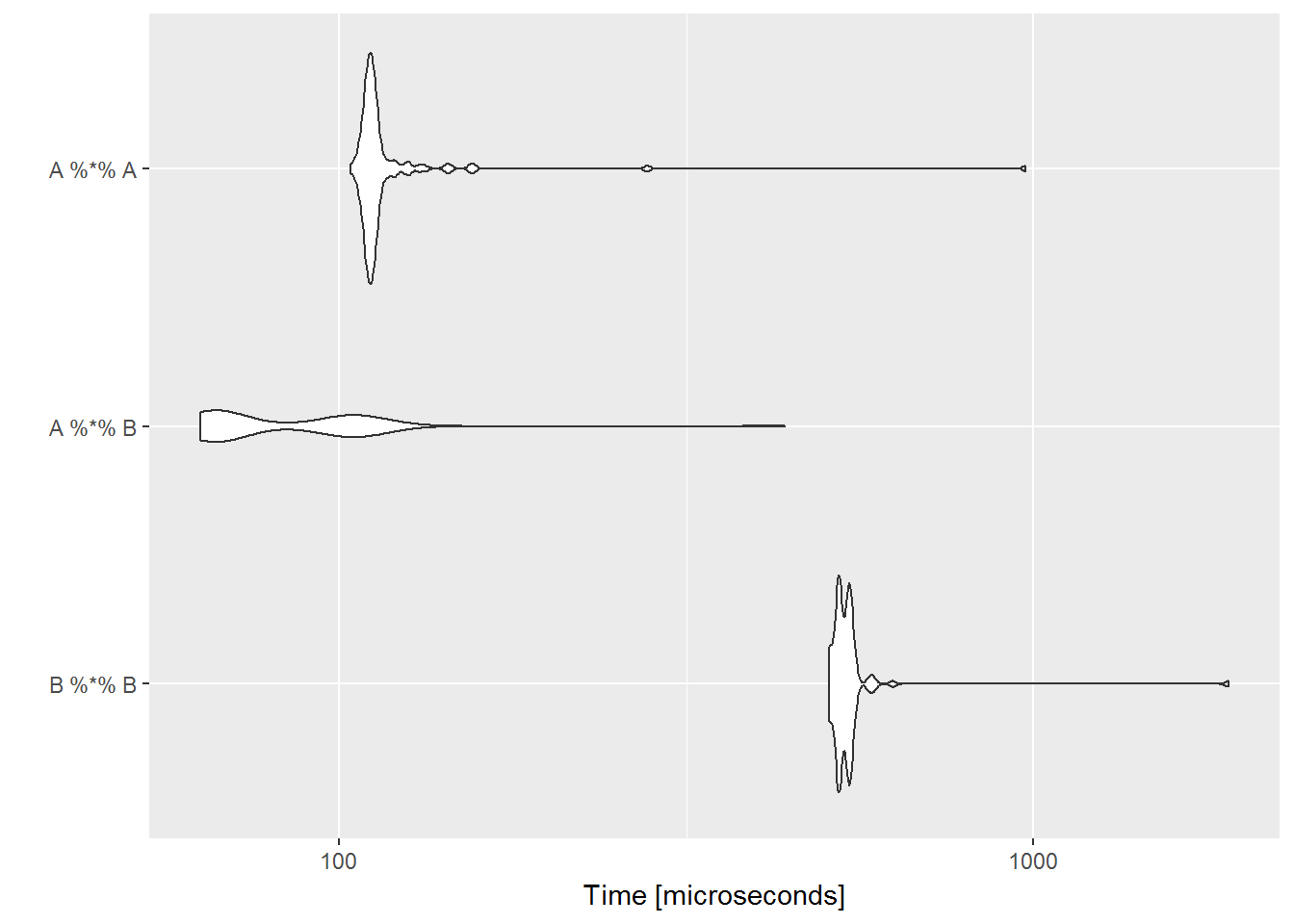However, your algorithm might involve adding matrices. The algorithms in MARSS involve many additions. Converting all the matrices to Matrix does not help since, A+B and A+A can be slow unless you are working with very large sparse matrices.

Another task that is slow is subsetting and if you need to assign a value to a subset of a sparse matrix, it is much, much slower.

res=microbenchmark(B[1,1],B[1,1]<-1,A[1,1],A[1,1]<-1,C[1,1],C[1,1]<-1, times=1000)
autoplot(res)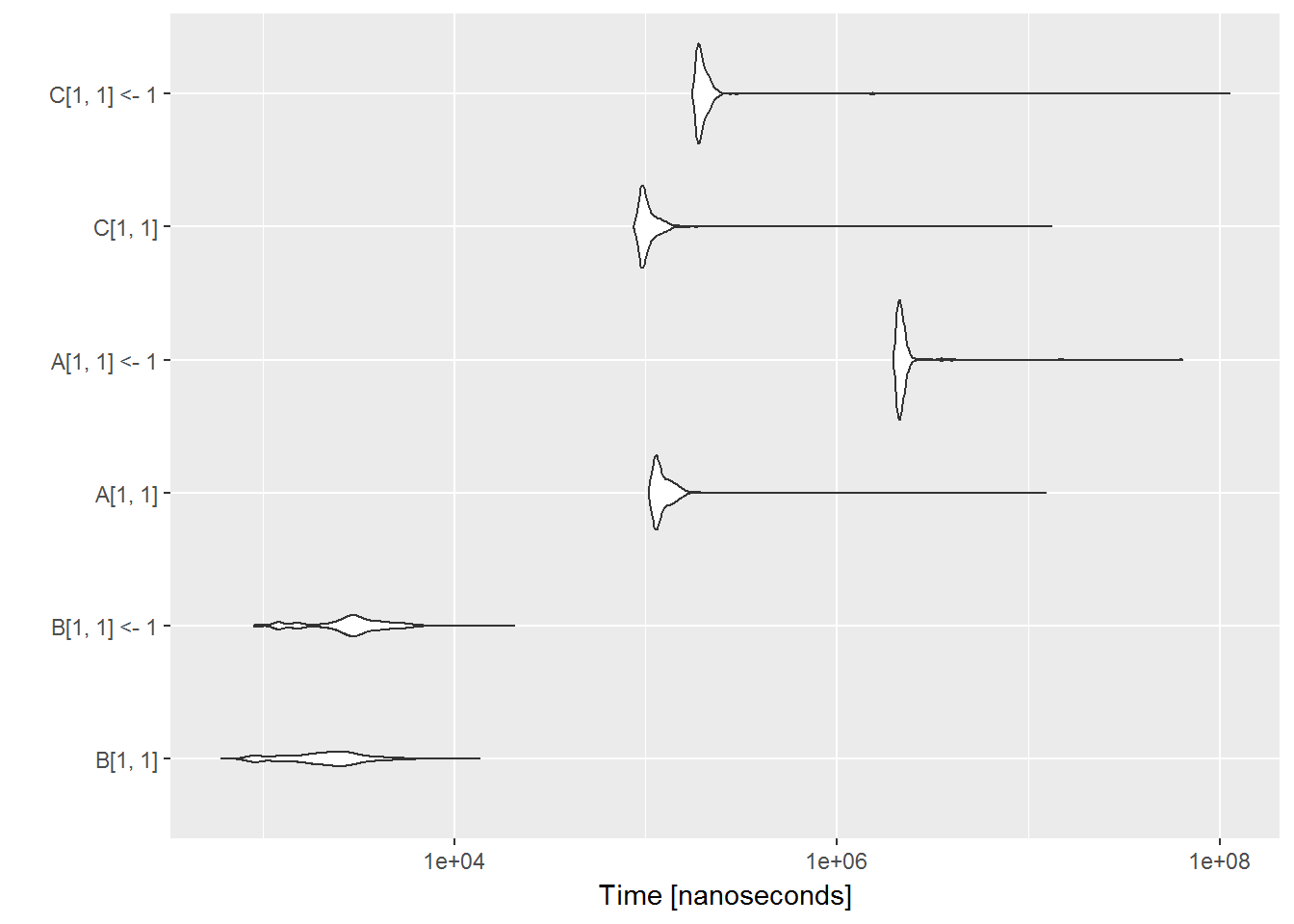One of the rules of fast R code is to never subset matrices or vectors. You should work on the whole vector or matrix in one step. However, there are cases where that is difficult and you might want or need to subset.

And another task that is slow is changing the dimensions, especially for sparse matrices.

n=100
A=Matrix(0, n, n) #dsCMatrix, sparse
B=as.matrix(A)
C=Matrix(1:n, n, n) #dgeMatrix, dense
res=microbenchmark(dim(B)<-c(n*n,1), dim(A)<-c(n*n,1),dim(C)<-c(n*n,1))
autoplot(res)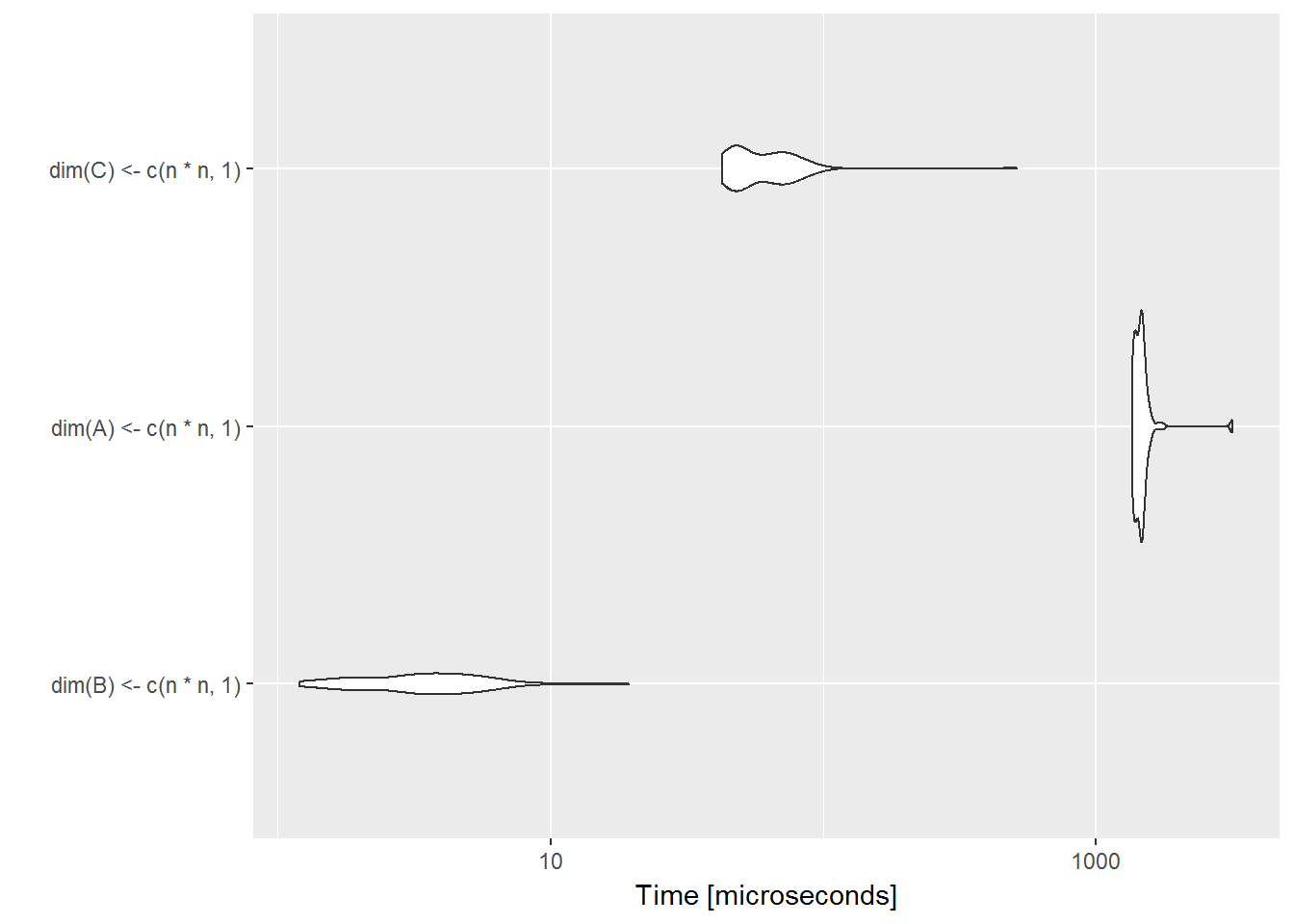One of the utility functions in MARSS, takes 3 matrices, subsets a column and does a little math:

parmat=function(f, d, par, r, c, t=1){
parvec=f[,t]+kronecker(t(par),diag(r*c))%*%d[,t]
parmat=parvec
dim(parmat)=c(r,c)
parmat
}
r=4; c=3; p=3; TT=10
f=Matrix(1,r*c,TT)
d=Matrix(0,r*c*p,TT)
par=Matrix(1,p,1)
ff=as.matrix(f); dd=as.matrix(d); ppar=as.matrix(par)
res=microbenchmark(parmat(ff,dd,ppar,r,c), parmat(f,d,par,r,c))
autoplot(res)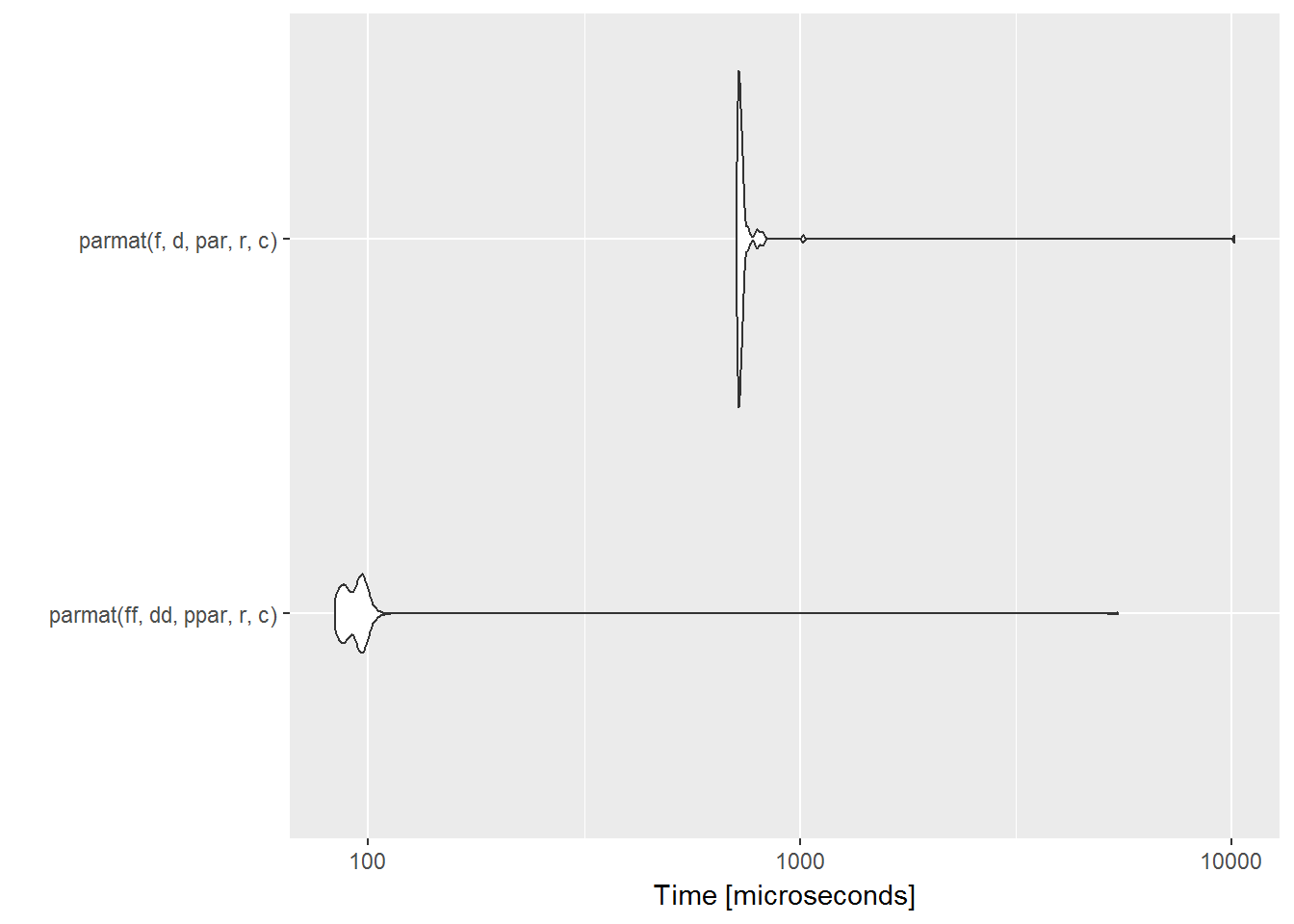This simple operation is very slow. Granted as r and c increase, the ff and dd matrices will become huge and we run out of memory. Thus the need for these matrices to be in sparse format—they are indeed highly sparse under normal circumstances.

A quick profile of parmat using say

library(profvis)
profvis({ for(i in 1:100) parmat(f,d,par,r,c) })

will show that the kronecker product is a bottleneck. So first thing we will do is get rid of that by rewriting the function as as we know what the form of f and d are:

parmat2=function(f, d, par, r, c, t=1){
p = dim(d)/dim(f)
D=d[,t]; dim(D)=c(r*c,p)
parvec=f[,t]+D%*%par
parmat=parvec
dim(parmat)=c(r,c)
parmat
}

parmat2 is much faster.

r=40; c=30; p=3; TT=10
f=Matrix(1,r*c,TT)
d=Matrix(c(0,1,0),r*c*p,TT)
par=Matrix(1,p,1)
ff=as.matrix(f); dd=as.matrix(d); ppar=as.matrix(par)
microbenchmark(parmat(ff,dd,ppar,r,c), parmat2(ff,dd,ppar,r,c), parmat(f,d,par,r,c), parmat2(f,d,par,r,c),times=1)
## Unit: microseconds
##                         expr        min         lq       mean     median
##   parmat(ff, dd, ppar, r, c) 185007.270 185007.270 185007.270 185007.270
##  parmat2(ff, dd, ppar, r, c)   6692.462   6692.462   6692.462   6692.462
##      parmat(f, d, par, r, c) 683513.684 683513.684 683513.684 683513.684
##     parmat2(f, d, par, r, c)    443.127    443.127    443.127    443.127
##          uq        max neval
##  185007.270 185007.270     1
##    6692.462   6692.462     1
##  683513.684 683513.684     1
##     443.127    443.127     1

A profile of parmat2

profvis({ for(i in 1:1000) parmat(f,d,par,r,c) })

indicates that the bottleneck is the subscripting. Subscripting a dgCMatrix is slow since the indices of the values is directly available. A dgCMatrix is in condensed column format for a sparse matrix.

We will create a dgCMatrix using sparseMatrix. If you use Matrix, then you do not know what class you will get. Use ?sparseMatrix to see how it works. You can specify a sparse matrix in triplet or condensed column format. The output can be either but default is condensed column. In triplet format, i are the rows of the non-zero values and j are the columns.

dgC=sparseMatrix(i=c(2,2,3), j=c(1,2,3), x=1)
dgC
## 3 x 3 sparse Matrix of class "dgCMatrix"
##
## [1,] . . .
## [2,] 1 1 .
## [3,] . . 1

This an S4 object. Let’s look at the slots.

str(dgC)
## Formal class 'dgCMatrix' [package "Matrix"] with 6 slots
##   ..@ i       : int [1:3] 1 1 2
##   ..@ p       : int [1:4] 0 1 2 3
##   ..@ Dim     : int [1:2] 3 3
##   ..@ Dimnames:List of 2
##   .. ..$: NULL ## .. ..$ : NULL
##   ..@ x       : num [1:3] 1 1 1
##   ..@ factors : list()

The i slot of this S4 object is the rows of each non-zero values reading down the columns, like what you passed to sparseMatrix except that the row numbering is like in matlab, row 1 is 0. So dgC@i is the i you passed in minus 1.

The p slot (dgC@p) shows the cummulative sum on non-zero values in each column. The p starts with a 0 always and then the cumulative sum starts so p is number of columns + 1. So p is

c(0, cumsum(colSums(dgC)))
##  0 1 2 3
dgC@p
##  0 1 2 3

The as(..., "TsparseMatrix") function is handy if you have a matrix in dgCMatrix format and you want it in triplet format so you can get the row/column indices of the non-zero values. Like you passed to sparseMatrix. The rows and column indices are in the i and j slots but in matlab format so row 1 is 0.

as(dgC, "TsparseMatrix")@i
##  1 1 2
as(dgC, "TsparseMatrix")@j
##  0 1 2